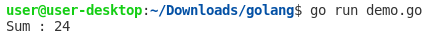## Posts

Showing posts with the label Slice

### How to find the sum of an Array/Slice of numbers in Go LanguageGiven an  Array  and  Slice  of Integers, Write a Go Language Program to find the sum of the elements of the  Array  and Slice . Note: Once an  Array  has allocated its size, the size can no longer be transmuted.  A  Slice  is a variable-length version of an  Array , providing more flexibility for developers. A range clause provides a way to iterate over an  Array   or  Slice. Example 1: Program to find the sum of the elements of an Array using for loop range package main import ( "fmt" ) func Sum(numb [ 6 ] int ) int { output := 0 for _, n := range numb { output += n } return output } func main() { num := [ 6 ] int { 5 , 1 , 7 , 2 , 3 , 6 } fmt.Println( "Sum :" , Sum(num)) } Output: Example 2: Program to find the sum of the elements of a Slice using for loop range package main import ( "fmt" ) func Sum(numb [] int ) int { output := 0 for _, n := range numb { output += n } return